# Gibbs Phase Rule

In each phase chemical potential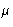is a function of P and T. Phase equilibrium condition: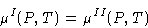This determines a line in P-T plane. Two lines can intersect--there three phases meet! (Triple point).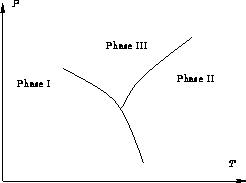In other words, two equations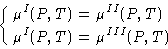have one isolated solution!

Binary mixtures: we add a new variable--concentration c and have two chemical potentials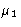and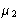. What is the largest number of phases to coexist?

If there are r phases, we have r+2 variables (P, T, cI, cII,...). There are 2r chemical potentials--2(r-1) equations. They have unique solution, if r+2=2(r-1), or r=4. In binary solutions four phases can coexist in a special point!

General case: n-component mixture, r phases. We have 2+r(n-1) variables for n(r-1) equations. Maximal value for r is 2+r(n-1)=n(r-1), or

r=n+2

This is Gibbs Phase Rule. The maximum number of phases in equilibrium is two plus the number of components.

© 1997 Boris Veytsman and Michael Kotelyanskii
Thu Oct 2 21:02:12 EDT 1997